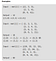# The Problem:

## Given a gold mine of n*m dimensions. Each field in this mine contains a positive integer which is the amount of gold in tons. Initially the miner is at first column but can be at any row. He can move only (right, right up ,right down) that is from a given cell, the miner can move to the cell diagonally up towards the right or right or diagonally down towards the right. Find out maximum amount of gold he can collect. (source: geeksforgeeks.org)Source: geekforgeeks.org

In simple words the problem boils down to the following points:

1. The miner starts at the first column, (any row). And after every step he moves one step right into the next column, until he reaches the final column.
2. Our job is to make the miner move through the path which gives him the maximum gold and return the maximum amount of gold collected.

## My Greedy Approach

So as usual, I thought why not just select the cell with the maximum value in the first column and then keep on moving right into the next column selecting the maximum value thereafter from the available choices (i.e right, right up and right down from the current cell).

But then I realized, okay what if there is a case where the first cell has a high value but after that all the other choices has very low values. See the below matrix for example:-

So here if you see, according to the greedy method, we will choose 10 first since it is the maximum in the first column. Then 1, 3, 4. This will give us a total value of 10+1+3+4 = 18.

But if you would have chosen 5 first, then 6,7,8 you will get a total value of 5+6+7+8 = 26. which is higher than the value we would have got through our greedy method. So clearly the greedy method I thought about is wrong.

But then I thought, okay so what if I calculated the maximum gold I get for all the values in the first column and then select the maximum one. That is, in the above example, I will select the first row, first column -> 10 first and then continue the path till the last column by selecting the maximum value thereafter out of the available 3 choices.

Then I will calculate the gold I will get for 2nd row, 1st column . Then 3rd row, 1st column until the last row, 1st column. I will store the values for each of its path and then output the maximum one.

I even wrote the whole code for this approach.

But unfortunately, THIS WAS WRONG. The code was correct but my whole approach was wrong.

I was doing one thing right and that is iterating through all the rows in the first column and then calculating the gold I will get through their paths. But I was still taking the MAXIMUM from the available 3 choices for all the other columns after the first column. So I was still doing the wrong Greedy approach for the rest of the columns. Below is an example where the above code fails:-

So the correct path here is

87–>99–>76->78->88->84->43->95 = 650.

But the above method outputs the following path:

87->99->94->60->95->70->39->78 = 622.

WRONG!!!!!!

# Dynamic Programming Solution

So I finally realized, okay I have to get back and look at the whole problem through a different angle. So I did just that, I put my laptop and slept.

Then I woke up, looked at it again and something wonderful struck my mind. The maximum gold I can get from a particular cell here, is not just the value of the cell, but the sum of the current value of the cell and the gold I will get after following the best path through there.

So take the 2nd row, 3rd column cell for example here with value 4. But the total gold I will get from that cell is not 4. It is 4+5=9.

But however the total gold for the cells in the last column will the values itself, because there is nowhere to go after the last column.

So what if starting from the last second column, we keep on replacing the values in the matrix by the total gold we will get from that particular cell.

So let me repeat the formula again:-

Maximum Gold from a cell = Current Value of Cell + maximum gold by following the best path OR maximum of the 3 choices in the next column.

But we have to remember to start from the last column and not the first.

Below is the code I used for the following approach:

So first 2 if statements are for special cases of Input where the matrix has only 1 row or 1 column.

Then you can see I simply iterated through all the columns in reverse and then through each of the rows in the columns, and updated the cell value using the formula above.

Then once it is complete, I simply iterated through all the cells in the first column and returned the maximum value.

And as expected this passed all the test cases. Hope I was able to let you have a view of the thought process I went through while solving this problem.

For more Dynamic Programming Problems and their solutions, you can check out my github repo here:- https://github.com/realdiganta/Dynamic-Programming

I build cool stuff… Sometimes weird too

## More from Diganta Kalita

I build cool stuff… Sometimes weird too